Courses

MCQ (Previous Year Questions) - Thermodynamics (Level 2)

25 Questions MCQ Test Chemistry for JEE Advanced | MCQ (Previous Year Questions) - Thermodynamics (Level 2)

Description
Attempt MCQ (Previous Year Questions) - Thermodynamics (Level 2) | 25 questions in 50 minutes | Mock test for JEE preparation | Free important questions MCQ to study Chemistry for JEE Advanced for JEE Exam | Download free PDF with solutions
QUESTION: 1

Solution:
QUESTION: 2

Which of the following statement is false ? [JEE 2001]

Solution: The work done depends on the path it follows to reach the final state in thermodynamics.
QUESTION: 3

Show that the reaction CO(g) +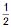O2(g) → CO2(g) at 300 K is spontaneous and exothermic, when the standard entropy is –0.094 kJ mol–1K–1. The standard Gibbs free energies of formation for CO2 and CO are –394.4 and –137.2 kJ mol–1, respectively. [JEE 2001]

Solution:
QUESTION: 4

Two moles of a perfect gas undergoes the following processes :                                                [JEE 2002]

(a) a reversible isobaric expansion from (1.0 atm, 20.0 L) to (1.0 atm, 40.0 L) ;

(b) a reversible isochoric change of state from (1.0 atm, 40.0 L) to (0.5 atm, 40.0 L) ;

(c) a reversible isothermal compression from (0.5 atm, 40.0L) to (1.0 atm, 20.0 L) ;

(i) Sketch with labels each of the processes on the same P-V diagram.

(ii) Calculate the total work (w) and the total heat change (q) involved in the above processes.

(iii) What will be the values of DU, DH and DS for the overall process ?

Solution:
QUESTION: 5

One mol of non-ideal gas undergoes change of state (2.0 atm, 3.0 L, 95 K) to (4.0 atm, 5.01, 245 K) with a change in internal energy (DU) = 30.0 L-atm. The change in enthalpy (DH) of the process in L-atm

[JEE 2002]

Solution:
QUESTION: 6

Two mole of an ideal gas is expanded isothermally and reversibly from 1 litre to 10 litre at 300 K. The enthalpy change (in kJ) for the process is [JEE 2004]

Solution:
QUESTION: 7

The enthalpy of vapourization of a liquid is 30 kJ mol–1 and entropy of vapourization is 75 J mol–1 K. The boiling point of the liquid at 1 atm is [JEE 2004]

Solution:
QUESTION: 8

One mole of a liquid (1 bar, 100 ml) is taken in an adiabatic container and the pressure increases steeply to 100 bar. Then at a constant pressure of 100 bar, volume decreases by 1 ml. Find DU and DH

[JEE 2004]

Solution:
QUESTION: 9

One mole of monoatomc ideal gas expands adiabatically at initial temp. T against a constant external pressure of 1 atm from one litre to two litre. Find out the final temp. (R = 0.0821 litre. atm K–1 mol–1):

[JEE 2005]

Solution:
QUESTION: 10

A process A → B is difficult to occur directly instead it takes place in the three successive steps.

DS (A → C) = 50 e.u.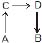DS (C → D) = 30 e.u.

DS (B → D) = 20 e.u. where e.u. is entropy unit.                                                                    [JEE 2006]

Then the entropy change for the process DS (A → B) is:

Solution:
QUESTION: 11

The molar heat capacity of a monoatomic gas for which the ratio of pressure and volume is one.

Solution:
QUESTION: 12

For the reaction.                                                                   [JEE 2006]

2CO(g) + O2(g)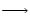2CO2(g) ; DH = _560 kJ mol–1

In one litre vessel at 500 K the initial pressure is 70 atm and after the reaction it becomes 40 atm at constant volume of one litre. Calculate change in internal energy. All the above gases show significant deviation from ideal behaviour. (1 L atm = 0.1 kJ)

Solution:
QUESTION: 13

For the process H2O(l) (1 bar, 373 K) → H2O (g) (1 bar, 373 K), the correct set of thermodynamic parametes is                                                                        [JEE 2007]

Solution:
QUESTION: 14

Statement-I : There is a natural asymmetry between converting work to heat and converting heat to work.Statement-II : No process is possible in which the sole result is the absorption of heat from a reservoir and its complete conversion into work

. [JEE 2008]

Solution:
*Multiple options can be correct
QUESTION: 15

Among the following, the state function(s) is (are)                                                                       [JEE 2009]

Solution:
QUESTION: 16

One mole of an ideal gas is taken from a to b along two paths denoted by the solid and the dashed lines as shown in the graph below. If the work done along the solid line path is ws and that along the dotted line path is wd , then integer closest to the ratio wd/ w5 is [JEE 2010]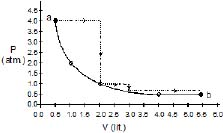Solution:
QUESTION: 17

Match the transformations in Column-I with appropriate options in Column-II.                                 [JEE 2011]

Column-I Column-II

(A) CO2(s)→CO2(g) (P) Phase transition

(B) CaCO3(s)→CaO(s)+ CO2(g) (Q) allotropic change

(C) 2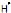→H2(g) (R) DH is positive

(D) P(white.solid)P(red, solid) (S) DS is positive

(T) DS is negative

Solution:
*Multiple options can be correct
QUESTION: 18

For an ideal gas, consider only P-V work in going from a initial state X to the final state Z. The final state Z can be reached by either of the two paths shown in the figure. Which of the following choice (s) is (are) correct ? [take DS as change in entropy and w as work done]                                                            [JEE 2012]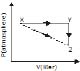Solution:
*Multiple options can be correct
QUESTION: 19

The reversible expansion of an ideal gas under adiabatic and isothermal conditions is shown in the figure. Which of the following statement(s) is are correct?                                                            [JEE 2012]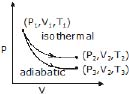Solution:
*Multiple options can be correct
QUESTION: 20

Benzene and naphthalene form an ideal solution at room temeprature. For this process the true statement(s) is (are)                                                                                              [JEE 2013]

Solution:
QUESTION: 21

A fixed 'm' of a gas is subjected to transformation of states from K to L M to N and back to K as shown in the figure [JEE 2013]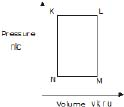The succeeding operations that enable this transformation of states are

Solution:
QUESTION: 22

A fixed 'm' of a gas is subjected to transformation of states from K to L M to N and back to K as shown in the figure [JEE 2013]The pair of isochoric processes among the transformatin of states is

Solution:
*Multiple options can be correct
QUESTION: 23

The figure below shows the variation of specific heat capacity (C) of a solid as a function of temperature (T). The temperature is increased continuously from 0 to 500 K at a constant rate. lgnoring any volume change, the following statement(s) is (are) correct to a reasonable approximation.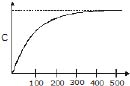Solution:
QUESTION: 24

One mole of a monatomic ideal gas is taken along two cyclic processes E→F→G→E and E→F→H→E as shown in the PV digram. The processes involved are purely isochoric, isobaric, isothermal or adiabatic.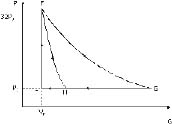Match the paths in List I with the magnitudes of the work done in List II and select the correct answer using the codes given below the lists.

List I List II

(P) G→E 1. 160 P0V0In2

(Q) G→H 2. 36 P0V0

(R) F→H 3. 24 P0V0

(S) F→G 4. 31 P0V0

Codes :

P Q R S

Solution:
QUESTION: 25

The standard enthalpies of formation of CO2(g), H2O(l) and glucose(s) at 25ºC are –400 kJ/mol, –300 kJ/mol and –1300 kJ/mol, respectively. The standard enthalpy of combustion per gram of glucose at 25ºC is

Solution:Use Code STAYHOME200 and get INR 200 additional OFF Use Coupon Code

Track your progress, build streaks, highlight & save important lessons and more!

Similar Content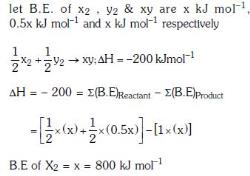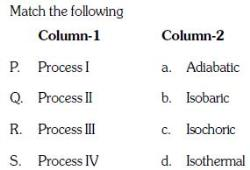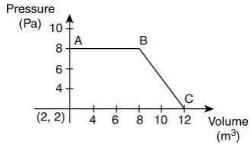Related tests